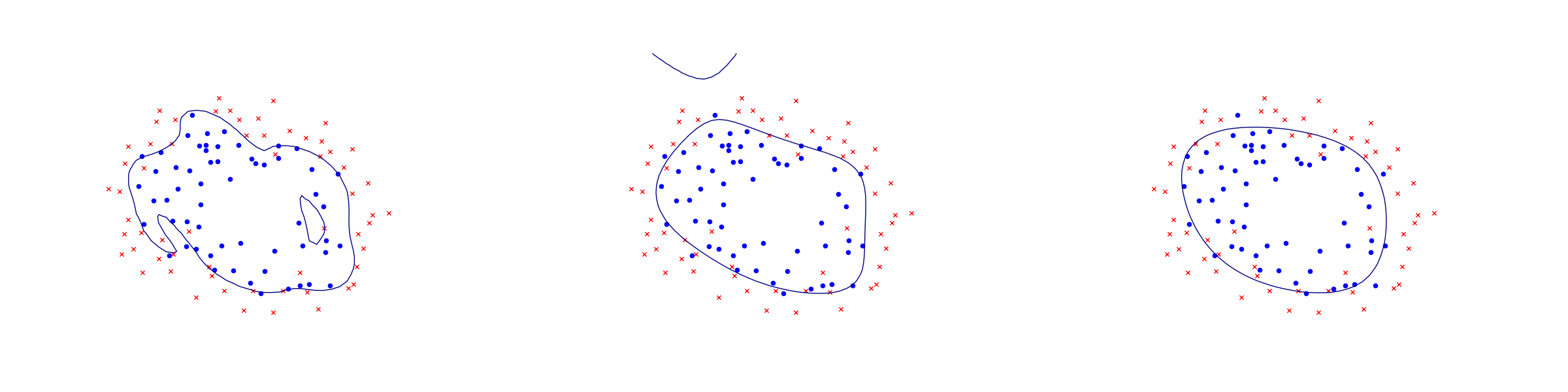# 11.5 Logistic regression¶

Logistic regression is an example of a binary classifier, where the output takes one two values 0 or 1 for each data point. We call the two values classes.

Formulation as an optimization problem

Define the sigmoid function

$S(x)=\frac{1}{1+\exp(-x)}.$

Next, given an observation $$x\in\real^d$$ and a weights $$\theta\in\real^d$$ we set

$h_\theta(x)=S(\theta^Tx)=\frac{1}{1+\exp(-\theta^Tx)}.$

The weights vector $$\theta$$ is part of the setup of the classifier. The expression $$h_\theta(x)$$ is interpreted as the probability that $$x$$ belongs to class 1. When asked to classify $$x$$ the returned answer is

$\begin{split}x\mapsto \begin{cases}\begin{array}{ll}1 & h_\theta(x)\geq 1/2, \\ 0 & h_\theta(x)<1/2.\end{array}\end{cases}\end{split}$

When training a logistic regression algorithm we are given a sequence of training examples $$x_i$$, each labelled with its class $$y_i\in \{0,1\}$$ and we seek to find the weights $$\theta$$ which maximize the likelihood function

$\prod_i h_\theta(x_i)^{y_i}(1-h_\theta(x_i))^{1-y_i}.$

Of course every single $$y_i$$ equals 0 or 1, so just one factor appears in the product for each training data point. By taking logarithms we can define the logistic loss function:

$J(\theta) = -\sum_{i:y_i=1} \log(h_\theta(x_i))-\sum_{i:y_i=0}\log(1-h_\theta(x_i)).$

The training problem with regularization (a standard technique to prevent overfitting) is now equivalent to

$\min_\theta J(\theta) + \lambda\|\theta\|_2.$

This can equivalently be phrased as

(11.20)$\begin{split}\begin{array}{lrllr} \minimize & \sum_i t_i +\lambda r & & & \\ \st & t_i & \geq - \log(h_\theta(x)) & = \log(1+\exp(-\theta^Tx_i)) & \mathrm{if}\ y_i=1, \\ & t_i & \geq - \log(1-h_\theta(x)) & = \log(1+\exp(\theta^Tx_i)) & \mathrm{if}\ y_i=0, \\ & r & \geq \|\theta\|_2. & & \end{array}\end{split}$

Implementation

As can be seen from (11.20) the key point is to implement the softplus bound $$t\geq \log(1+e^u)$$, which is the simplest example of a log-sum-exp constraint for two scalar variables $$t,u$$. This is equivalent to

$\exp(u-t) + \exp(-t)\leq 1$

and further to

(11.21)$\begin{split}\begin{array}{rclr} (z_1, 1, u-t) & \in & \EXP & (z_1\geq \exp(u-t)), \\ (z_2, 1, -t) & \in & \EXP & (z_2\geq \exp(-t)), \\ z_1+z_2 & \leq & 1. & \end{array}\end{split}$
Listing 11.9 Implementation of $$t\geq \log(1+e^u)$$ as in (11.21). Click here to download.
// t >= log( 1 + exp(u) ) coordinatewise
void softplus(Model::t      M,
Expression::t t,
Expression::t u)
{
int n = (*t->getShape());
auto z1 = M->variable(n);
auto z2 = M->variable(n);
M->constraint(Expr::hstack(z1, Expr::constTerm(n, 1.0), Expr::sub(u,t)), Domain::inPExpCone());
M->constraint(Expr::hstack(z2, Expr::constTerm(n, 1.0), Expr::neg(t)), Domain::inPExpCone());
}


Once we have this subroutine, it is easy to implement a function that builds the regularized loss function model (11.20).

Listing 11.10 Implementation of (11.20). Click here to download.
// Model logistic regression (regularized with full 2-norm of theta)
// X - n x d matrix of data points
// y - length n vector classifying training points
// lamb - regularization parameter
std::pair<Model::t, Variable::t>
logisticRegression(std::vector<std::vector<double>> & X,
std::vector<bool>                & y,
double                           lamb)
{
int n = X.size();
int d = X.size();       // num samples, dimension

Model::t M = new Model();

auto theta = M->variable(d);
auto t     = M->variable(n);
auto reg   = M->variable();

M->constraint(Var::vstack(reg, theta), Domain::inQCone());

auto signs = std::make_shared<ndarray<double,1>>(shape(n), [y](ptrdiff_t i) { return y[i] ? -1 : 1; });

softplus(M, t, Expr::mulElm(Expr::mul(new_array_ptr<double>(X), theta), signs));

return std::make_pair(M, theta);
}


Example: 2D dataset fitting

In the next figure we apply logistic regression to the training set of 2D points taken from the example ex2data2.txt . The two-dimensional dataset was converted into a feature vector $$x\in\real^{28}$$ using monomial coordinates of degrees at most 6.Fig. 11.6 Logistic regression example with none, medium and strong regularization (small, medium, large $$\lambda$$). Without regularization we get obvious overfitting.¶